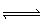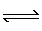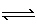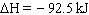Name:    Practice Test 1A

Multiple Choice
Identify the letter of the choice that best completes the statement or answers the question.

1.

Which of the following sets of factors affect the value of the rate constant, k?
 A) reactant concentration and temperature B) reactant concentration and surface area C) activation energy and reactant concentration D) temperature and activation energy

2.

The equilibrium constant, Kp, for the reaction below  is 55.2 at 425°C.  A rigid cylinder at this temperature contains 0.127 atm of hydrogen, 0.134 atm of iodine and 1.055 atm of HI.  Which of the following statements is correct?
H2 (g)  +  I2 (g)2 HI (g)
 A) The forward reaction must proceed to establish equilibrium B) The system is at equilibrium C) The reverse reaction must proceed to establish equilibrium D) more data is required to draw a conclusion

3.

At 500°C the equilibrium constant, Kp, is 4.00 x10–4 for the equilibrium:

2 HCN (g)H2 (g)   +  C2N2 (g)

What is Kp for the following reaction?

H2 (g)   +   C2N2 (g)2 HCN (g)
 A) 4.00 x 104 B) –4.00 x 10–4 C) 2.00 x 10–4 D) 2.50 x 103

4.

When the reaction AB + C is studied, a plot of log [A] vs. time gives a straight line with a negative slope.  The order of this reaction is
 A) zero B) first C) third D) second

5.

The decomposition of hydrogen peroxide is a first order reaction with a rate constant of 1.06x10–3 min–1.  How long will it take for the concentration of H2O2 to drop from 0.0200M to 0.0120M?
 A) 4550 min B) 7.55 min C) 31400 min D) 482 min

6.

For the reaction H2 (g) + I2 (g)2 HI (g), at 700 K, Kc= 56.6.  If an equilibrium mixture at 700 K was found to contain 0.55 M HI and 0.21 M H2, then the I2 concentration must be
 A) 0.046 M B) 0.21 M C) 22 M D) 0.025 M

7.

Nitrogen dioxide can dissociate into nitric oxide and oxygen:

2 NO2 (g)2 NO (g) + O2 (g)Under which reaction conditions would you expect to produce the largest amount of oxygen?
 A) high temperature, high pressure B) high temperature, low pressure C) low temperature, high pressure D) low temperature, low pressure

8.

In which of the following equilibriums would the a change in volume have no effect?
 A) N2 (g)  + 3 H2 (g)2 NH3 (g) B) NO (g) + O3 (g)NO2 (g) + O2 (g) C) N2 (g)  + 2 O2 (g)2 NO2 (g) D) N2O4 (g)2 NO2 (g)

9.

Which factor will not increase the concentration of ammonia as represented by the equilibrium below?

3 H2(g)  +   N2 (g)2 NH3 (g)A) increasing the concentration of N2 B) increasing the pressure C) increasing the concentration of H2 D) increasing the temperature

10.

The rate law for the reaction 3A2B is rate = k [A] with a rate constant of 0.0447 hr–1.  What is the half-life of the reaction if the initial concentration of A is 0.500 M?
 A) 6.25 hr B) 15.5 hr C) 0.0224 hr D) 44.7 hr

11.

Considering the equilibrium shown below, which of the following changes will increase the
concentration of HgI42–?
HgO (s)  +  H2O (l)HgI42– (aq)  +  OH (aq)
 A) increasing the concentration of OH– B) increasing mass of HgO C) adding a catalyst D) adding 6M HNO3

12.

The decomposition of N2O5 has an activation energy of 102 kJ/mol andH= +55 kJ/mol.  What is the activation energy for the reverse reaction?
 A) 55 kJ/mol B) 157 kJ/mol C) 47 kJ/mol D) 102 kJ/mol

13.

A catalyst is effective because
 A) it lowers the activation energy for the reaction. B) it increases the number of collisions between the molecules. C) it increases the temperature of the molecules in the reaction. D) it increases the number of molecules with enrgy equal to greater than the activation energy.

14.

For the reaction  A + 2 B2 C + 2 D, the following data were collected.  Determine the rate law for this reaction.

 Exp [A], M [B], M Rate, M/min 1 0.125 0.200 7.25 2 0.375 0.200 21.75 3 0.250 0.400 14.50 4 0.375 0.400 21.75

 A) Rate = k [A][B] B) Rate = k [A] C) Rate = k [A][B]3 D) Rate = k [A]2[B]

15.

Reaction intermediates differ from activated complexes in that
 A) they are stable molecules with normal bonds and are frequently isolated. B) they are unstable and can never be isolated. C) they are intermediate structures which have characteristics of both reactants and products. D) they are molecules with normal bonds rather than partial bonds and can occasionally be isolated.